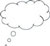# 2 Developing an understanding of fractions

The second activity focuses on students physically representing the concepts of fractions. This is also called embodiment. You will ask them to use their bodies to represent mathematical ideas. If the students move themselves to make fractions of a whole, they will begin to develop their concept of what a fraction is and how they can work with fractions.

Before attempting to use the activities in this unit with your students, it would be a good idea to complete all, or at least part, of the activities yourself. It would be even better if you could try them out with a colleague as that will help you when you reflect on the experience. Trying them for yourself will mean you get insights into a learner’s experiences, which can, in turn, influence your teaching and your experiences as a teacher.

## Activity 2: Physically representing fractions

### Preparation

First create a space, and ask eight students to come to the front of the class or somewhere where the rest of the class can see them.

### The activity

• Ask someone else to divide the group into half.
• Reform the rectangle, then ask another student to divide the group in half in a different way.
• Ask the students what is the same and what is different about the new half of the group.
• Now ask another student to divide the eight students into quarters (fourths). Again ask whether there is a different way to do this division, and what is the same and what is different about the new way of dividing into quarters.
• Now change the number of students and go through the process above again. It may be that dividing into quarters is difficult but depending on the chosen number, continue asking for ,, and so on, until a fraction that cannot be done is reached. Ask the students why you cannot find that fraction of these students. Dividing one student into bits is not allowed!
• Ask the students to work in groups of 12. You could appoint a leader in each group to note down ideas if the class does not split evenly into groups of 12. Ask them to work out all the fractions they can divide 12 students into.Video: Using questioning to promote thinking

## Case Study 1: Mrs Rawool reflects on using Activity 1

This is the account of a teacher who tried Activity 1 with his elementary students.

First, I invited eight students to come to the front of the class and to form themselves into a rectangular shape where the rest of the class could see them. I then asked student Anoushka to come and divide these eight students in half, which was easy to do.

I then asked the class if the group of eight students could be divided in half in another way. This proved to be a little challenging, as the students were used to mathematics questions having just one answer, so they wondered at first if Anoushka was wrong. They needed clarification about what ‘different’ meant here. Of course, whichever way they divided the students in half, there were always four students in each half. Since this was the answer I was looking for, I gave them time to talk about these ideas.

Next, I asked student Nita to come to the front and divide the group into quarters. This time the students were able to suggest different ways to achieve this, and they were happy there would always be two students in each part.

I then asked another group of students to come to the front, this time with six students. This time I asked them to divide themselves into half in two ways. I asked ‘Do you always get the same answer?’ ‘Yes sir!’ they said. Then I asked ‘What other fraction can you divide yourselves into?’ They tried to divide themselves into quarters but they could not, but what they did find was that they could divide themselves into three parts and discussed what this fraction was called.

I then put the class into groups of 12 and asked them what fractions they could embody in their groups. One group came up with twelfths, but most worked happily on halves, quarters, thirds and sixths.

## Reflecting on your teaching practice

When you do such an exercise with your class, reflect afterwards on what went well and what went less well. Consider the questions that led to the students being interested and being able to progress, and those you needed to clarify. Such reflection always helps with finding a ‘script’ that helps you engage the students to find mathematics interesting and enjoyable. If they do not understand and cannot do something, they are less likely to become involved. Use this reflective exercise every time you undertake the activities, noting as Mrs Rawool did some quite small things that made a difference.Pause for thought Good questions to trigger such reflection are: How did it go with your class? What responses from students were unexpected? Why?What questions did you use to probe your students’ understanding? Did you feel you had to intervene at any point? What points did you feel you had to reinforce?Did you modify the task in any way? If so, what was your reasoning for this?

1 What’s so difficult about fractions?